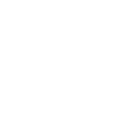×### FA Part 2 Mathematics Online Test

Here you can prepare FA Part 2 Mathematics Online Test. Click the button for FA Part 2 Mathematics 100% free full practice test.

## 12th Class Mathematics MCQ Test With Answer for Mathematics Full Book

Here at this page students will find the online test of Mathematics subjects of FA class. All the students are to inform that these online test system can be the true oath of their good practice. A good practice level is the warranty of good performance in the examination. Obviously, the students will perform well in the examination system will get exceptional FA Part II Result. Students are to inform that Online Test System students can find with chapter wise sequence and full Book Online Test are also available here. The test is presented in the form of multiple choice questions or MCQs.

This is the first time going to happen that students are provided with an online test system. This is all happened by the dedicated team of ilmkidunya.com. It is the platform which is totally concerned with the education system of Pakistan. The team of this platform assists students on every single level of education. 12th class students can also get FA class Past Papers or Mathematics Subject Past Papers for exceptional practice. A complete guide throughout the study session students get.

First of all, students get a safe Admission Guide. After getting safe admission students are provided with Online Study Resources. All those students who are preparing themselves for final examination are to inform that they will be able to get their FA Class Date Sheets here. Moreover, in the end, students will also get FA Class Result here on this page. Students are suggested to keep in touch with us for all updates. At the same time, students are also requested to share these helpful contents to your friends.

FA Part 2 Mathematics Test### 12th Class Mathematics MCQ Test With Answer for Mathematics Full Book

Sr. # Questions Answers Choice
1 (1, 0) is the solution of inequality :
• A. 7x + 2y &lt; 8
• B. x - 3y &lt; 0
• C. 3x + 5y &gt; 6
• D. -3x + 5y &gt; 2
2
• A.
• B. 0
• C. 4
• D. 7
3
• A. Constant
• B. Implicit
• C. Explicit
• D. Inverse
4
• A. Ellipse
• B. Parabola
• C. Hyperbola
• D. Circle
5 Non-vertical lines divide the plane into___________half plane:
• A. Upper and lower
• B. Many
• C. Left and Right
• D. None of these
6 There are _______ ordered pairs that satisfy the inequality ax + by &gt; c.
• A. Finitely many
• B. Two
• C. Infinitely many
• D. Four
7
• A. Position vector
• B. Null vector
• C. Unit vector
• D. None of these
8 If equation of circle is (x - h)<sup>2</sup> + (y - k)<sup>2</sup> = r<sup>2</sup>, then center of a circle:
• A. (-h, -k)
• B. (h, k)
• C. (-h, k)
• D. (h, -k)
9 If y is an image of x under the function f, we denote it by:
• A. x = f(y)
• B. x = y
• C. y = f(x)
• D. f(x, y) = c
10 The function f(x) = 3x<sup>2</sup> has minimum value at :
• A. x = 3
• B. x = 2
• C. x = 1
• D. x = 0

Sort By:
X

to continue to ilmkidunya.comFill the form. Our admission consultants will call you with admission options.

X

to continue to ilmkidunya.com

X

to continue to ilmkidunya.com

X